# post .pdf

### File information

Original filename: post.pdf

This PDF 1.5 document has been generated by LaTeX with hyperref package / pdfTeX-1.40.17, and has been sent on pdf-archive.com on 22/09/2016 at 23:53, from IP address 73.174.x.x. The current document download page has been viewed 448 times.
File size: 88 KB (5 pages).
Privacy: public file

post.pdf (PDF, 88 KB)

### Document preview

Post 1
Is there an easy (without much math) explaination about how a
frequency analysis is done?
Sure, but allow me a little bit of math to demonstrate the concept. If
you don’t understand it, try skipping to the next block of text.
You’re performing a harmonic frequency analysis, where the potential
energy of a one-dimensional harmonic oscillator is given by
1
E = kx2 ,
2
the potential at a given point is
∂E
,
∂x
and the force (or gradient) is the negative of the potential (a matter of
convention, it varies)
V =

F = −V = −kx,
which hopefully you recognize as Hooke’s law. Now, we are interested in
solving for k, the force constant, which is directly related to the frequency of
the system by
s

k
.
m
Mathematically, to get the force constant k from our original energy equation, differentiate one more time to give
ν=

∂ 2E
.
∂x2
This is where I’ll leave out the most of the gory details, particularly the
constant prefactors, but clearly we are not interested in the one-dimensional
problem, but a 3N -dimensional problem, because that’s how many atoms
there are. The equation now looks like
k=

1
∂ 2E
Hij = √
mi mj ∂xi ∂xj
where i, j run over all 3N Cartesian coordinates and Hij is the massweighted Hessian, the eigenvalues of which give the force constants. Phew.
1

From my NWChem output it seems like it’s going through every
atom in the molecule and does 6 calculations for each atom (labeled 1(+), 1(-), 2(+), 2(-), 3(+) and 3(-)) with energy gradients
for each atom as result. What is the program doing with each
atom (putting into 6 different states?) and how does it get the
frequencies from that?
Now, one thing I neglected to mention above is where the energy expression in the derivative comes from. The very first equation is only used to help
define how one would get the force constants. The energy is the quantum
chemical chemistry; this is the most general form, so it could be HartreeFock, DFT, MP2, CCSD, you name it. These quantum chemical methods
have well-defined energy expressions, which in theory can be differentiated
twice with respect to nuclear displacements. This results in a closed-form
equation which can be solved exactly, but in the case of wavefunction methods this expression is usually very large, so implementing it is very difficult
and computationally tends to require a large amount of memory. You may
have noticed that for HF and most DFT calculations the frequency part
doesn’t do all these other calculations, because the exact derivative expression is simple enough that it can be coded up. The alternative is to recognize
that
!

∂E
∂ 2E
=
∂xj ∂xi
∂xj ∂xi
which is the reason why all that expository math from before is useful. If no exact 2nd derivative is available, but a 1st derivative is, then the
2nd derivative can be obtained by analytically calculating the 1st derivative
(gradient) at finite difference points, where the other derivative is taken by
displacing a nuclear coordinate. Most programs have MP2 or RI-MP2 and
CCSD analytic gradients, but not analytic (exact) frequencies. The +/- is
because central difference is being performed, not forward as you might have
learned in school. When you read about ”numerical frequencies” in the literature, this is what’s being performed. In the case of CCSD(T) frequencies,
almost always only energies are available, so finite difference needs to be
performed along two coordinates, which usually leads to hundreds of energy
calculations.
So, all 3N Cartesian coordinates are being displaced forwards and backwards, and because the gradient can be represented as a giant vector, all of
these vectors can be arranged into the H matrix above.
2

Are there books explaining things like that (geometry optimization, frequency analysis and so on) for chemists?
Yes. The text I most strongly recommend is actually Exploring Chemistry
with Electronic Structure Methods. Older copies are, uh, easy to find, the
explanations are clear, and the examples are practical. Even if you don’t
use Gaussian most of it is very transferable to other packages. Think of
it as a workbook. Another one, which is short but dense is Jan Jensen’s
Molecular Modeling Basics, also meant for practitioners. Chris Cramer’s
book and Frank Jensen’s books are both good, but might be more than you
want. David Young’s book is very broad in scope while being short, so it
might leave you wanting more detail.
Another resource that I’ll plug is the Chemistry Stack Exchange, where
more involved questions like this fit in nicely, though I’m a bit biased since
I’m always hoping for more computational chemistry questions there.

Post 2
Just to check if I understand correctly: I need the force constants
(and mass) to calculate frequencies. Therefore I need the second
derivative of the potential energy in every of my 3xN dimensions.
So my MP2 calculation is able to calculate first derivatives (gradients) analytically but for the second derivative it uses the finite
difference method (why the central one and not forward or backwards? would cut the calculations in half) where it does one step
forward and one step backward for each dimension (so it moves
each atom a little bit +x, then -x, then +y,....) and calculates
again the gradients, and with the +, the - and the original gradient it can form the second derivative.
Bingo.
Central difference is more accurate than forward or backward, especially
in the case that the PES is not symmetric around the expansion point f (x),
which forward and backward difference assume. Analogously, consider the
different edges in the rectangle rule for numerical integration. One would
expect the midpoint rule to be slightly more accurate than using either of
the corners.
3

Now there’s one point I don’t get I think. My system has 3xN
dimensions, so a x,y and z position of each atom. Now the first
derivative of this are the (energy) gradients and it’s still a 3xN
matrix, right? This basically tell me how the energy of that
whole system changes if I move a certain atom into x, y or z
direction. Now the second derivatives would still be 3xN system
which would tell me how the change in change in energy (the
change in the gradient) is if I move a certain atom in a certain
direction. So if I do the second derivative for atom 1 in x direction
I need as a result a single value. But what I get is the change
in gradients not only for that one atom but for (potentially) all
atoms. So what does it use for that one value? The Eigenvalue
of that whole 3xN matrix?
The potential energy is being differentiated with respect to nuclear coordinates; the coordinates themselves are never differentiated, which would
place ’x’ in the numerator of the derivative. Because there are 3N nuclear coordinates, every time you differentiate you produce a quantity that contains
an extra dimension of size 3N. The potential energy is rank 0 (a scalar), the
gradient is rank 1 (a [3N ] vector, usually shown as a [N, 3] or [3, N ] matrix),
the Hessian is rank 2 (a [3N, 3N ] matrix), the cubic force constants are a
rank 3 (a [3N, 3N, 3N ] tensor), and so on.
By extension of what you said about gradients, the Hessian tells you how
the energy changes if you displace one of the 3N coordinates and then another
of the 3N coordinates. Because of how derivatives work (that last equality
I showed), it is perfectly valid to think of this as how the gradient changes
with respect to a further displacement.
The confusing thing might be you calculate all elements of the gradient
in a single shot. Assume that we’re doing forward finite difference, and we
have the gradient at the stationary point. As mentioned above, it’s best to
think of the gradient as a vector, even though it isn’t printed in the output
that way (it can be reshaped without mathematical consequence). Calculate
, where h is the step
1(+), then form the finite difference expression (1(+)−1(0))
h
size. This is d/dx1 of a quantity that is [3N ] in shape/size. There are 3N of
them that can be arranged as rows in the matrix.
Central finite difference is the same principle, except there’s 6N gradient calculations as the finite difference equation requires two new quantities
rather than one.
4

The eigenvalues are found only after all the matrix elements have been
calculated. The vector of eigenvalues is the diagonal representation of the
Hessian, and the eigenvectors correspond to the normal mode displacements.
And if I understand this correctly I dould do geometry optimization by calculating the gradients for every 3xN dimensions and
then move each atom in the direction the gradient points to until all the gradients are basically 0, which would mean I’m in
a (lokal) minima of potential energy? (this would explain why
optimization for optimization with fixed atoms the gradients for
those atoms were just set to 0 during opt)
Aside from some algorithmic stuff, this is exactly how geometry optimizations work. With regard to the constrained optimization, what packages do
is set up a Lagrange multiplier for every frozen coordinate to act as a penalty
function during the optimization so that a step is never taken along that coordinate, which could be real-space (a Cartesian translation) or internal (a
bond stretch, a torsion rotation, ...).

5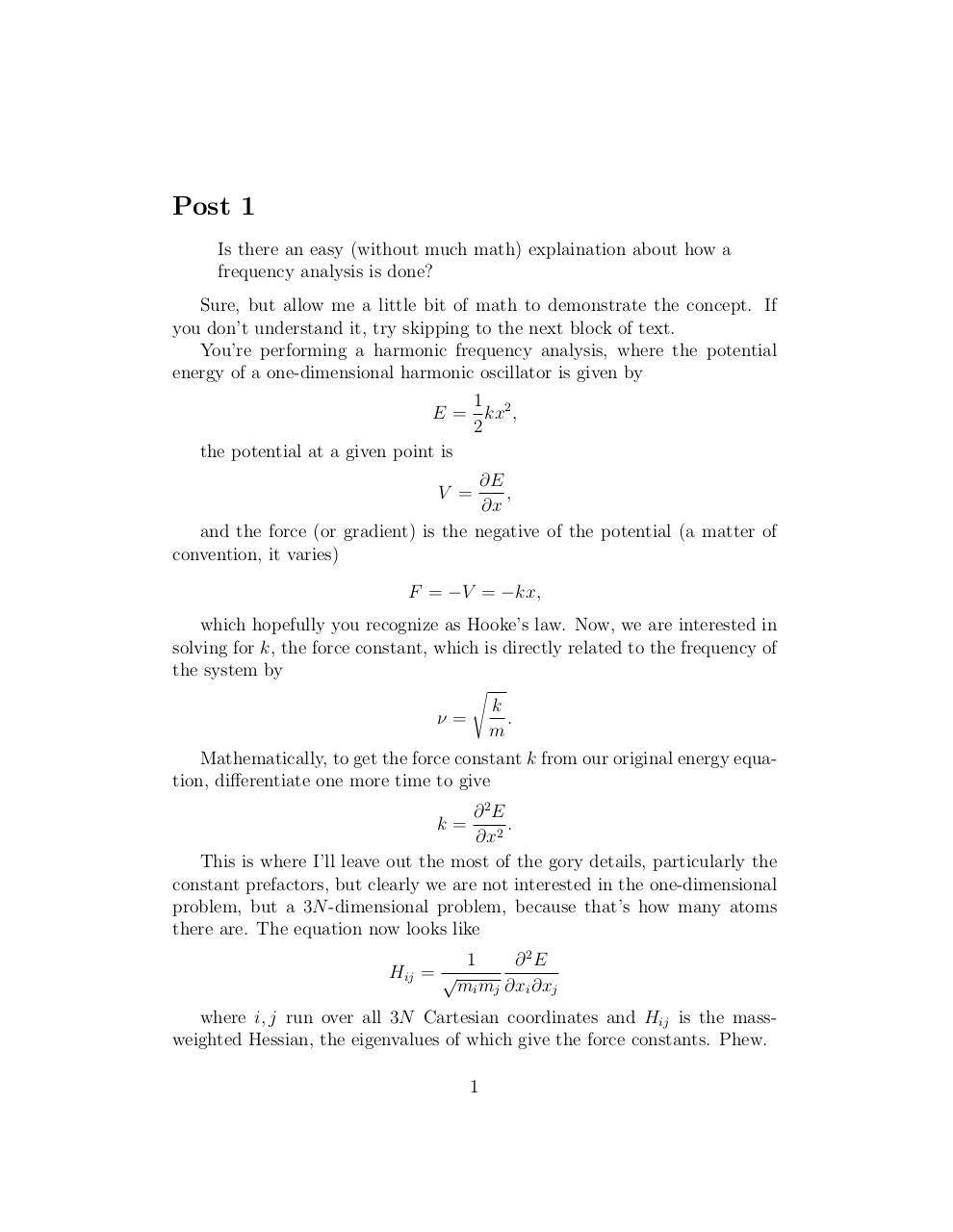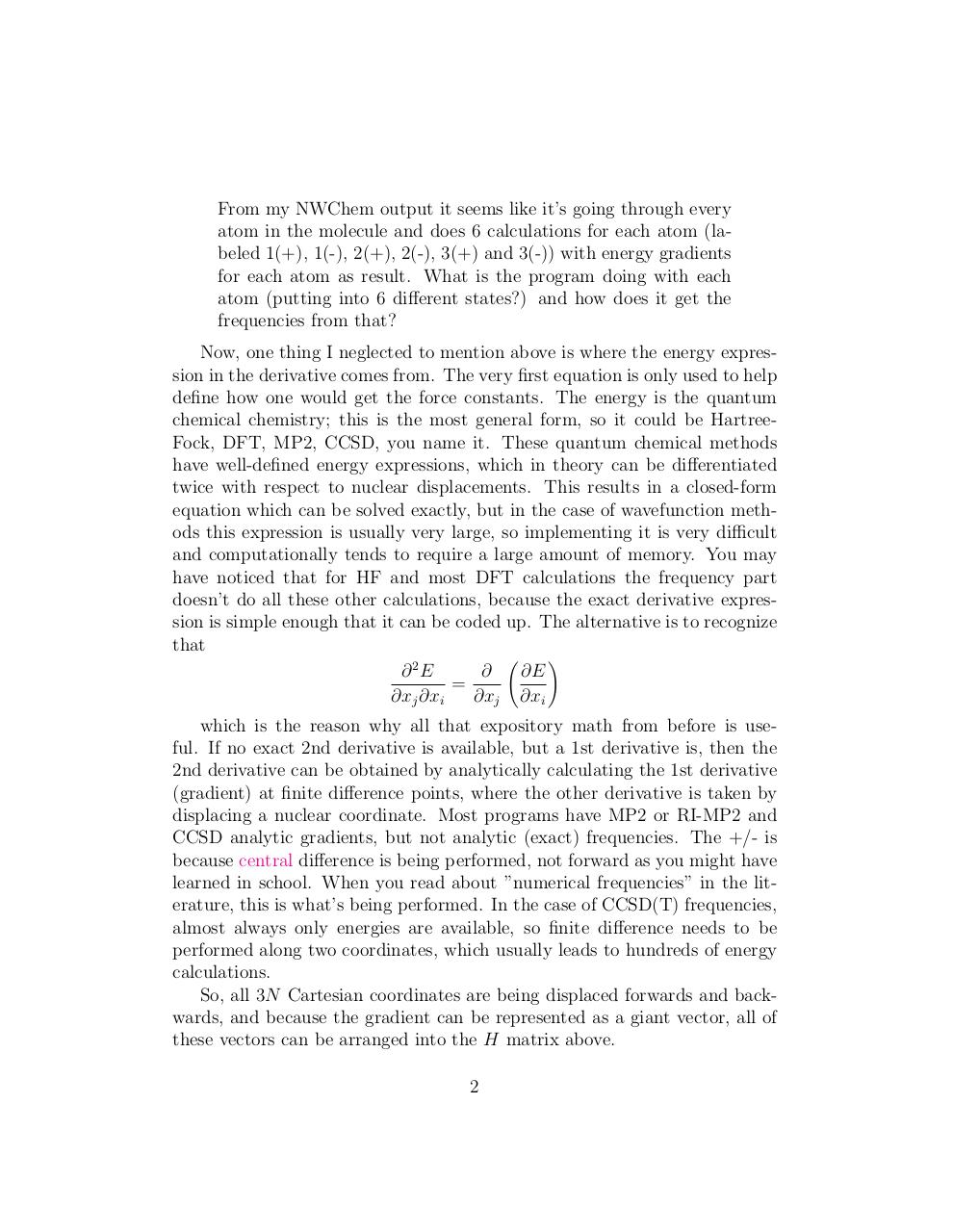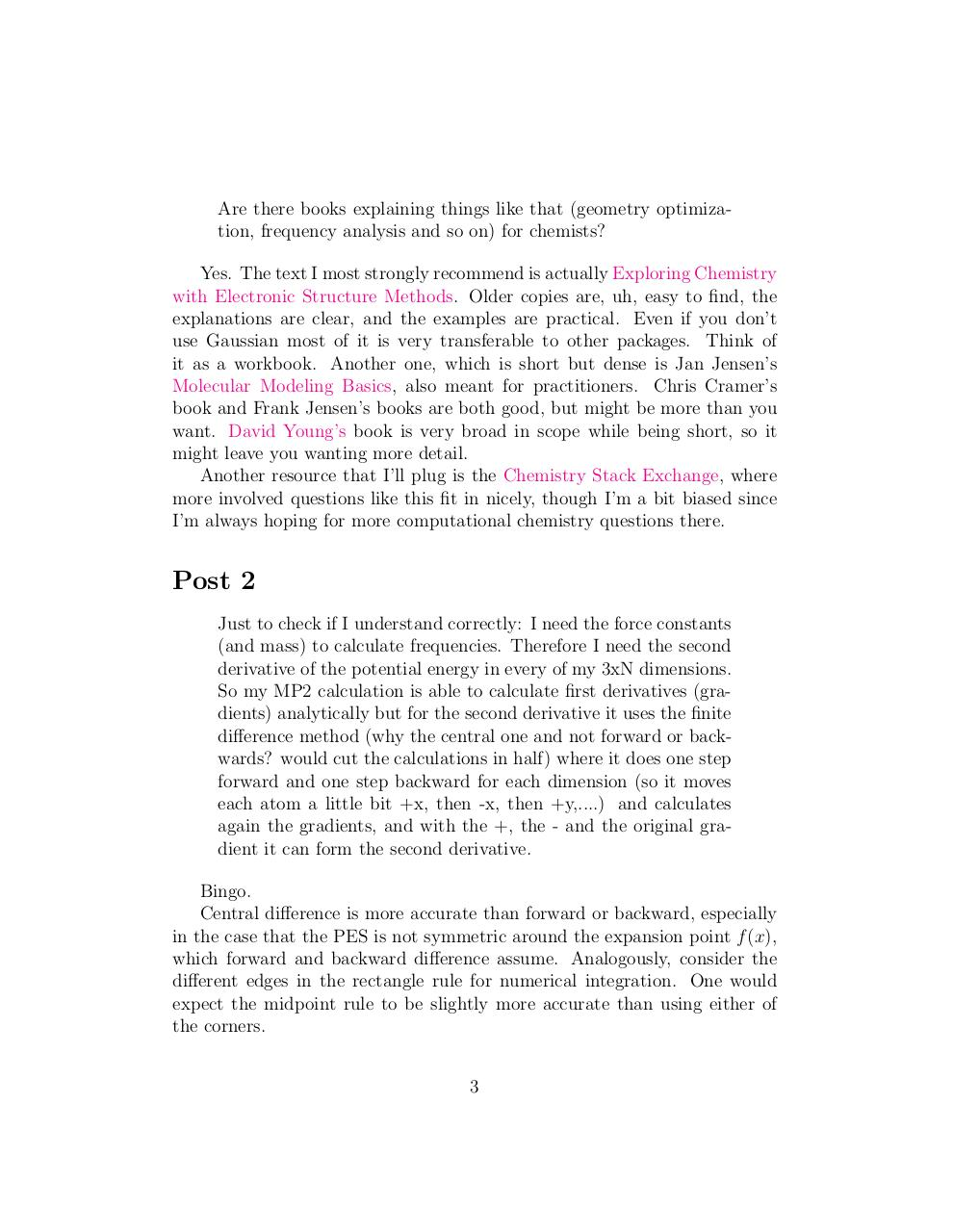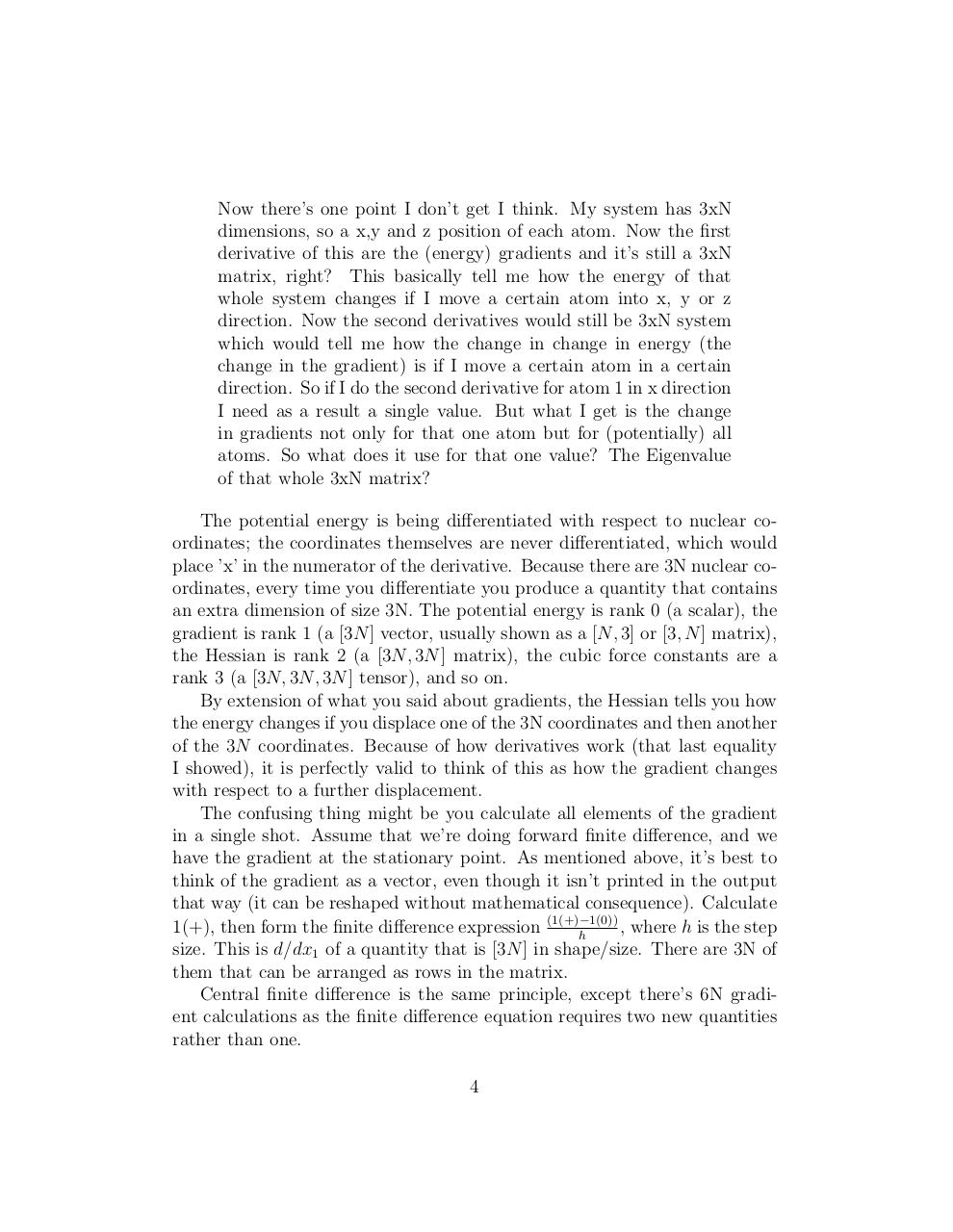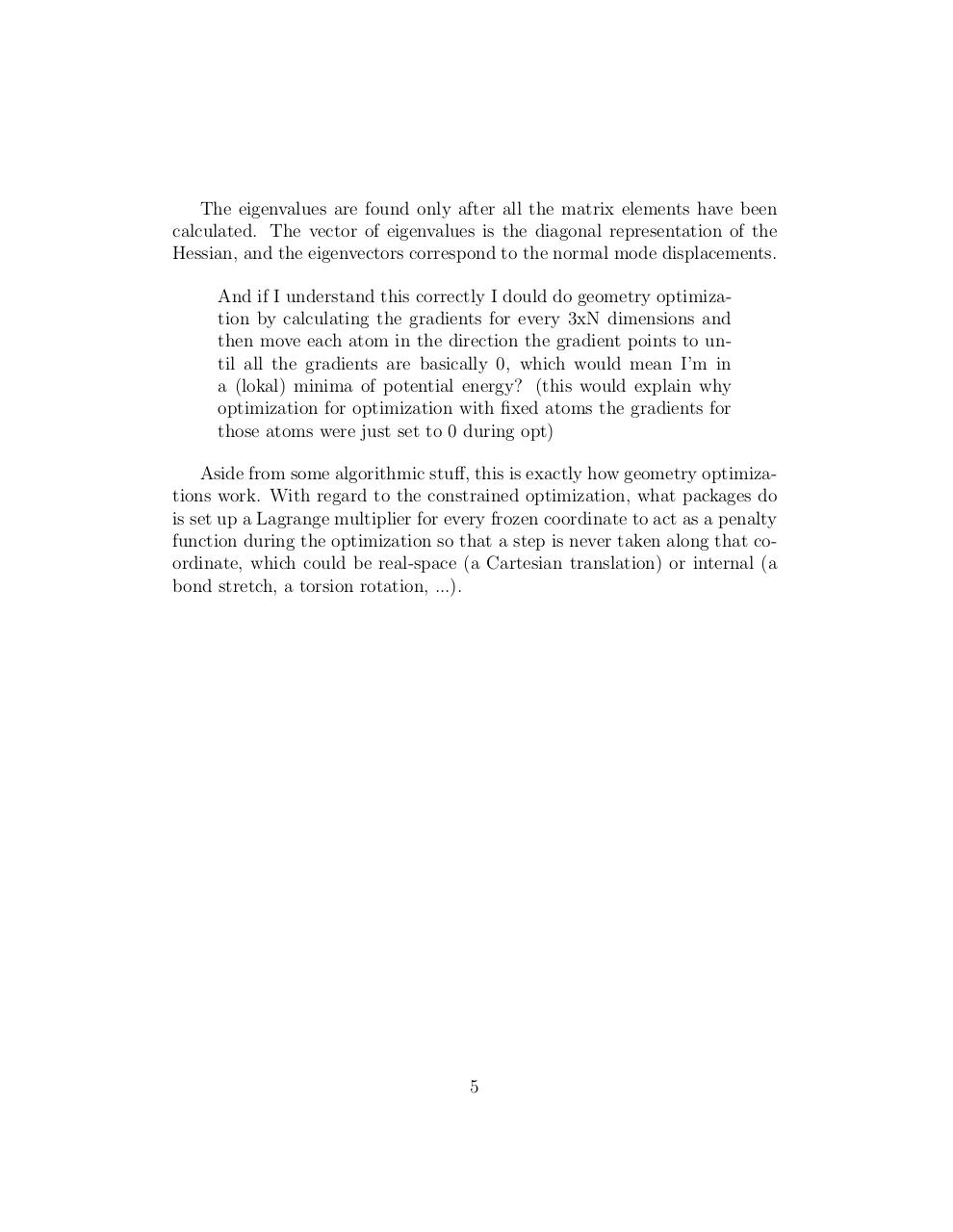#### HTML Code

Copy the following HTML code to share your document on a Website or Blog

#### QR Code### Related keywords